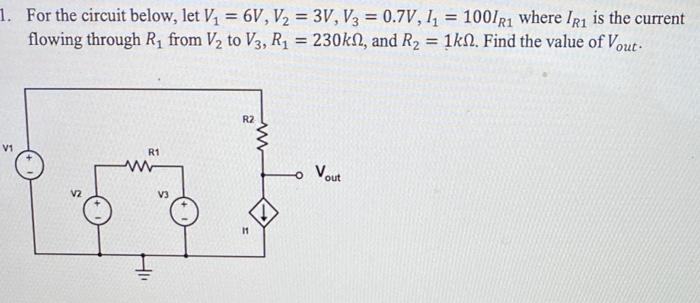Home / Expert Answers / Electrical Engineering / for-the-circuit-below-let-v-1-6-mathrm-v-v-2-3-mathrm-v-v-3-0-7-mathrm-v-i-1-pa232

# (Solved): For the circuit below, let $$V_{1}=6 \mathrm{~V}, V_{2}=3 \mathrm{~V}, V_{3}=0.7 \mathrm{~V}, I_{1 ...For the circuit below, let \( V_{1}=6 \mathrm{~V}, V_{2}=3 \mathrm{~V}, V_{3}=0.7 \mathrm{~V}, I_{1}=100 I_{R 1}$$ where $$I_{R 1}$$ is the current flowing through $$R_{1}$$ from $$V_{2}$$ to $$V_{3}, R_{1}=230 \mathrm{k} \Omega$$, and $$R_{2}=1 \mathrm{k} \Omega$$. Find the value of $$V_{\text {out }}$$.

We have an Answer from Expert